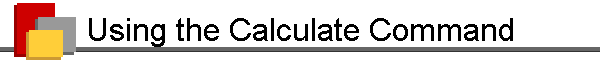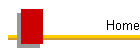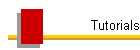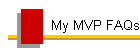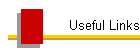# Better than an Easter egg: Using ToolsCalculate

This article was written for Word 2003 and earlier; for its application to Word 2007 and above, see the final section.

Among Word’s many undocumented features are some legacies from previous versions. One of these is the ToolsCalculate command. In Word 2.x for Windows and 5.1 for Mac, the Tools menu included a Calculate item that permitted users to perform calculations on selected numbers. The result was displayed in the status bar and also copied to the Clipboard, whence it could be pasted into the document.

Word 6.0 introduced the “formula field” (an expansion of the “expression field” that had already existed in Word 2.0), which greatly extended Word’s ability to perform calculations. Not limited to simple arithmetic operators, formula fields can use a variety of other functions. But formula fields are sometimes overkill, and they have their limitations. Although they are not entirely confined to tables, that is where they are easiest to use. And one of the most commonly used formula fields, { = SUM(ABOVE) }, not only is confined to tables but has some serious limitations even there: it stops adding at the first empty cell, and in Word 97 it can’t sum more than 85 rows accurately.

These limitations (and unfamiliarity with formula fields) were enough to send Word 6.0 users looking for the handy Calculate command, only to find it gone from the Tools menu. A Microsoft Knowledge Base article, “WD6X: Calculate Command Does Not Appear on the Tools Menu [Q120093]” (now retired), acknowledged its absence and advised users that “This change in the menu structure is by design in Word 6.0. To perform a calculation in Word 6.0, use the Formula command on the Table menu or run the ToolsCalculate macro from the Tools Macro dialog box.” Alas, the ToolsCalculate macro itself had disappeared by Word 95.

But all is not lost! ToolsCalculate is still available (in Word 2003 and earlier) through Tools | Customize. On the Commands tab, select All Commands in the Category list, then scroll down the Commands list until you find ToolsCalculate. Drag it to the menu bar and hover the mouse over “Tools” until the Tools menu drops down. Drop Calculate somewhere on the menu and begin to discover its usefulness:

1. You can use it anywhere. If you have constructed a simple table using tabs, you can select one column of numbers by holding down the Alt key while you drag. Then use the Calculate command. The status bar briefly displays the text “The result of the calculation is x,” and if you press Ctrl+V, the result will be inserted in your document. You can even sum a series of numbers in running text, such as 1, 2, 3, 4 or 1 2 3 4; just select them and run Calculate.

2. If you use Calculate in a table, you can sum a column (any number of rows, including empty cells—unlike SUM(ABOVE)), a row, or the entire table!

3. Calculate is not limited to sums, either. By using simple arithmetic operators, you can perform the following operations: addition, subtraction, multiplication, division, calculating a power, calculating a root, and calculating a percentage.

Following are excerpts from the instructions contained in the Microsoft Word User's Guide for Word 2.0.

## Adding with the Calculate Command

The math feature is especially useful if you want to add numbers in table columns, in table rows, or in columns created with tabs. Just select the row or column, and then choose Calculate from the Tools menu. Word calculates the result for you, and you can insert the result in a document.

Word ignores text and reads only numbers when calculating a selection. You don’t need to type a plus sign (+), because Word automatically adds selected numbers separated by spaces unless you specify another mathematical operation.

## Other Operations with the Calculate Command

You can also use the Calculate command to subtract, divide, or multiply numbers or to calculate percentages, powers, and roots. When you perform calculations other than addition, you need to include mathematical operators. For example, if you want to multiply two numbers, type the first number, an asterisk (*), and the second number. Select the numbers and the asterisk, and then choose Calculate from the Tools menu. Word performs the calculation and displays the result for a few seconds in the status bar. The result is also automatically copied to the Clipboard.

The following table shows the operators to use for various calculations.

Use

To

Example

Expression

Result

- or ( )

Subtract

Budget for the rest of the month

1500-976
or
1500 (976)

 524

/

Divide

Cost per month

\$7000/12

 \$583.33

*

Multiply

Rent per year

12*\$583

 \$6996.00

+ or space

Joint income

19,000+16,500
or
19,000 16,500

 35,500

%

Calculate percentage

Taxes due

\$155.79*6%

 \$9.35

^

Calculate power

Six squared

6^2

 36

^

Calculate power

Cube root of eight

8^(1/3)

 2

Note As shown in the preceding table, you can specify that a number be subtracted by enclosing it in parentheses. This is standard practice for many financial statements where numbers are listed in columns. In the following example, Word subtracts 125 from 500, and then adds 250.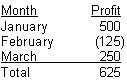### To perform math calculations:

1. Type the numbers you want to calculate, along with the appropriate mathematical operators.

Word can calculate numbers in a line, in a column or row in a table, or in a column separated from other columns with tabs. Word can also calculate numbers interspersed throughout the text in one or more paragraphs if you include a space on either side of each number.
2. Select the numbers and operators.

3. From the Tools menu, choose Calculate.

Word calculates the result and displays it for a few seconds in the status bar. The result is stored on the Clipboard.
4. To insert the result into your document, position the insertion point and choose Paste from the Edit menu (CTRL+V) or click the Paste button on the Toolbar.

## Guidelines for Calculation

Word uses the following guidelines when performing calculations:

• Word reads only numbers and operators when calculating a selection, ignoring text.

• Word uses ordered operations, meaning that certain calculations are performed before others. Percentage operations (%) take place before power and root (^) calculations. Power and root operations take place before multiplication and division (* and /). Multiplication and division take place before addition and subtraction (+ and -).

• Word calculates expressions in parentheses first. Enclosing numbers and an operator within parentheses tells Word to perform the enclosed operation first. However, enclosing a single number within parentheses tells Word to subtract it from the total. For example, if you select 25 (5*2), Word calculates a result of 35. But if you select 25 (5), Word calculates a result of 20.

• If one of the numbers in the selection contains a separator such as a comma or decimal point, the result also contains that separator. Make sure you have used the International [Regional Settings or Regional and Language Options] category of the Control Panel to select the appropriate list separator before you use the Calculate command.

• If one of the numbers in the selection contains the currency symbol set in the Control Panel, the result contains that currency symbol. Make sure you have used the [Regional Settings or Regional and Language Options] category of the Control Panel to select the appropriate currency symbol before you use the Calculate command.

• If one of the numbers in the selection contains a decimal fraction, the result includes the same number of decimal places. Word automatically adds two decimal places to the result if any of the numbers contains the currency symbol set in the Control Panel, or if the result includes a fraction. For example, the result of £75-25 is £50.00. Word displays the result of 7000/6 as 1166.67.

## Calculate in Word 2007 and above

Word 2007 and above can use the Calculate command in exactly the same ways, and it is still available in the Customize dialog (Office Button | Word Options | Customize | All Commands | Calculate in Office 2007; File | Options | Quick Access Toolbar | All Commands | Calculate in Word 2010 and above). As in previous versions, it has no button icon, but, unlike previous versions, Word 2007 and above don’t offer the ability to create a custom button icon. This is not a serious drawback when adding it to a menu, but Word 2007 and above have no menus, so it will have to be added to the Quick Access Toolbar (QAT), where it will be represented by the generic green ball. In Word 2010 and above, it can be added to the Ribbon; the command to look for at File | Options | Customize Ribbon is Tools Calculate. If you prefer to add a keyboard shortcut, again look for ToolsCalculate in the Customize Keyboard dialog.

If you have added this button in a previous version, you can import it into Word 2007 or above; see Word MVP Graham Mayor’s article “Toolbars and Word 2007.” You can design your own button icon or use the one available in my article “Custom Toolbar Buttons.”